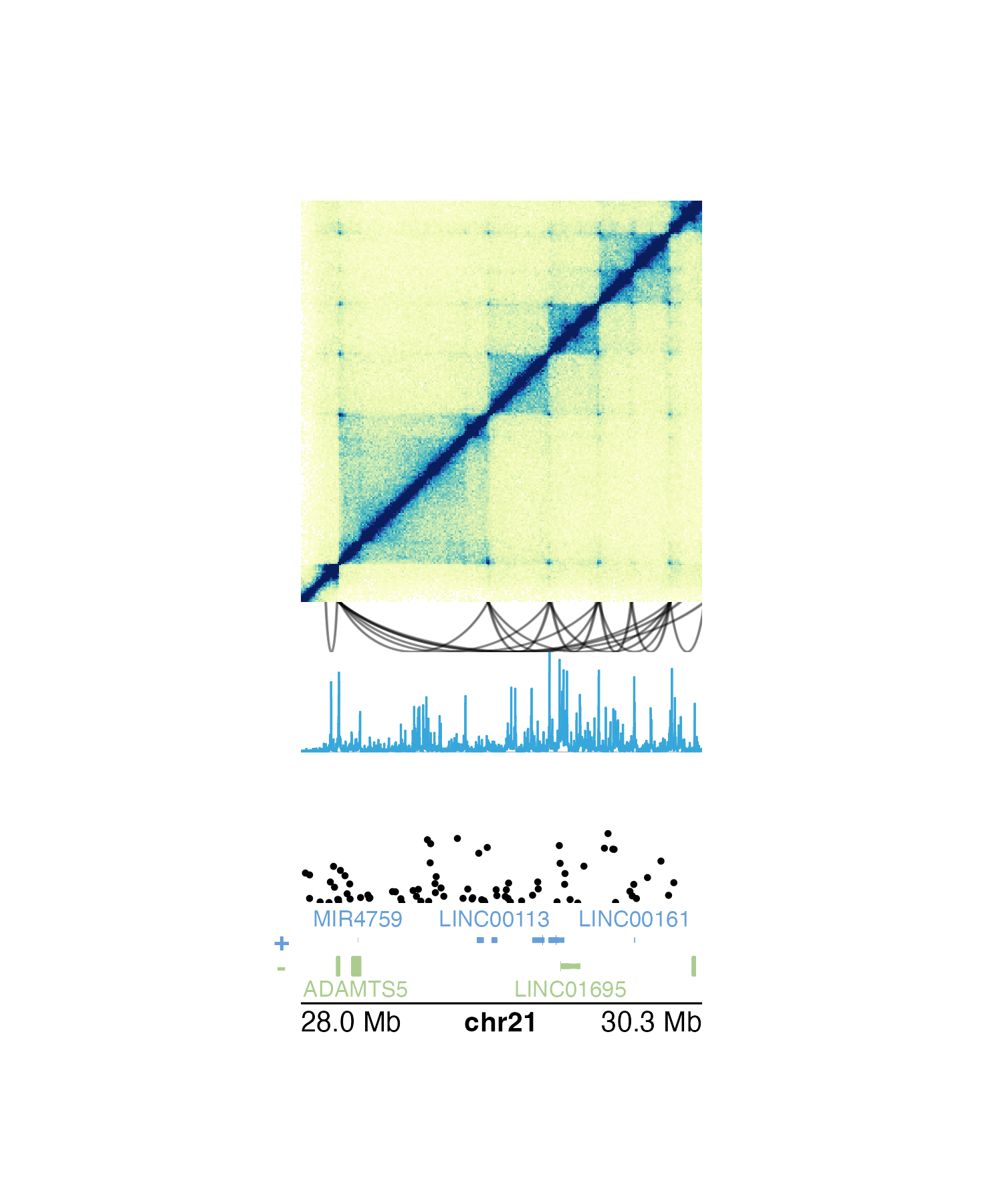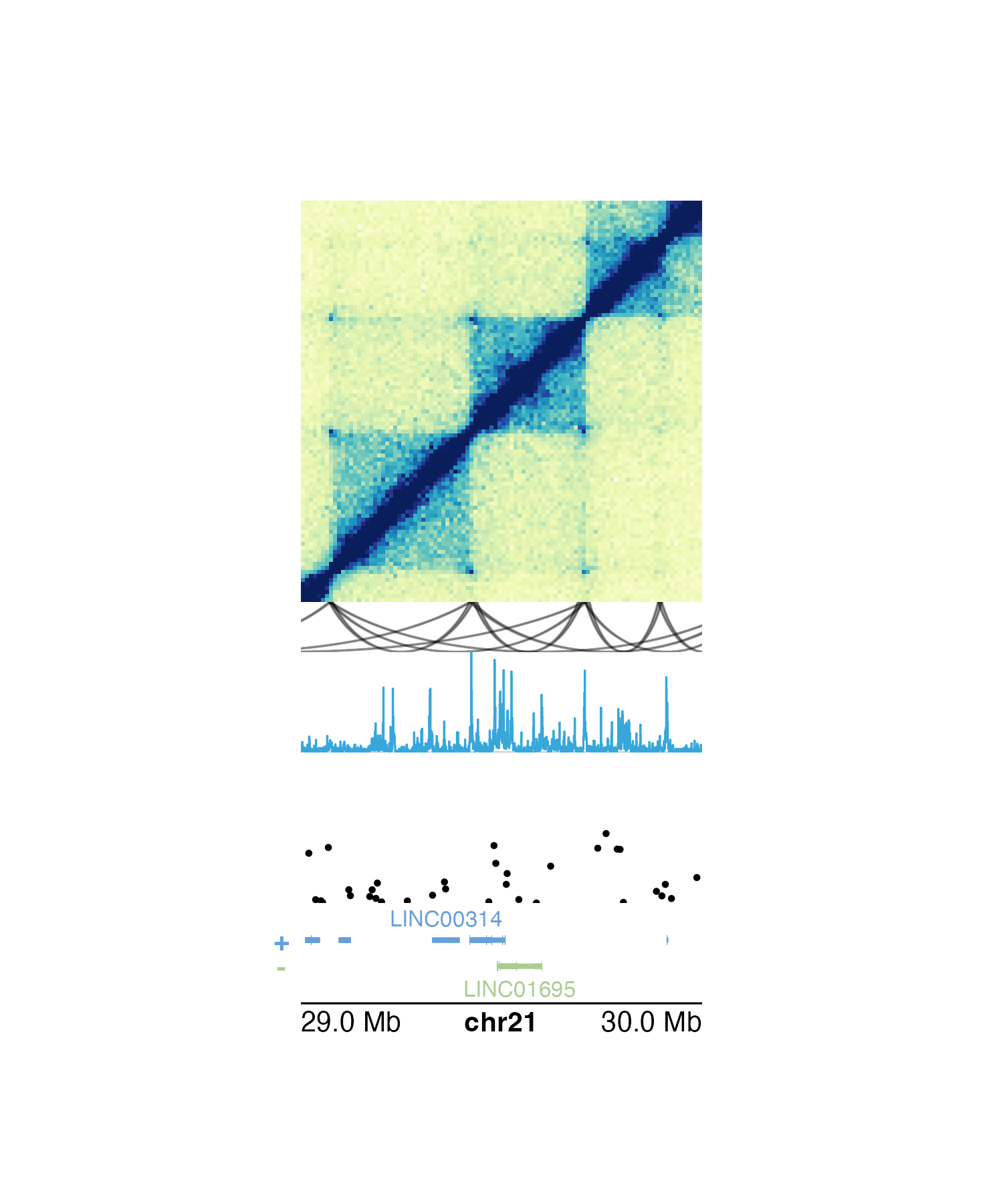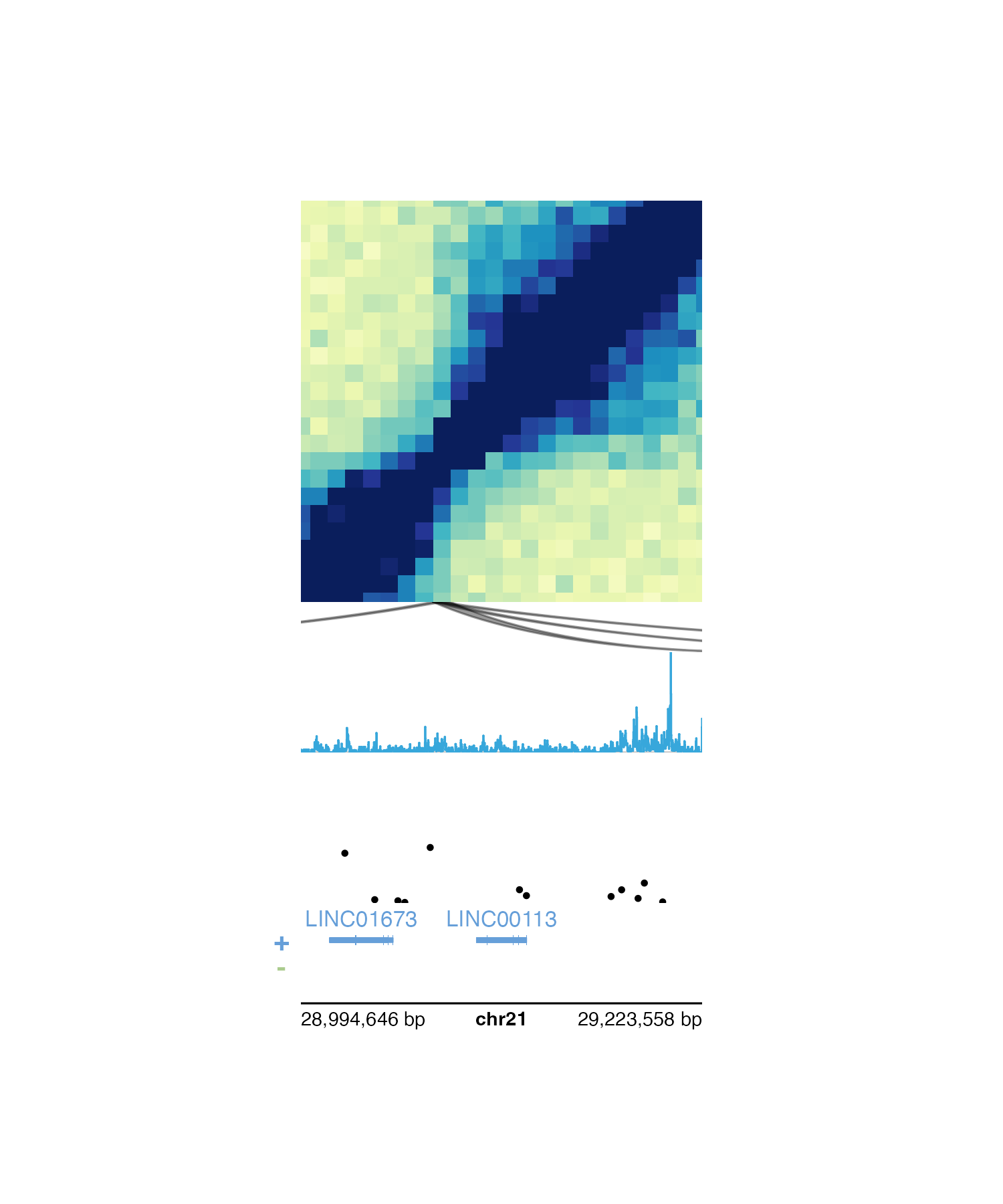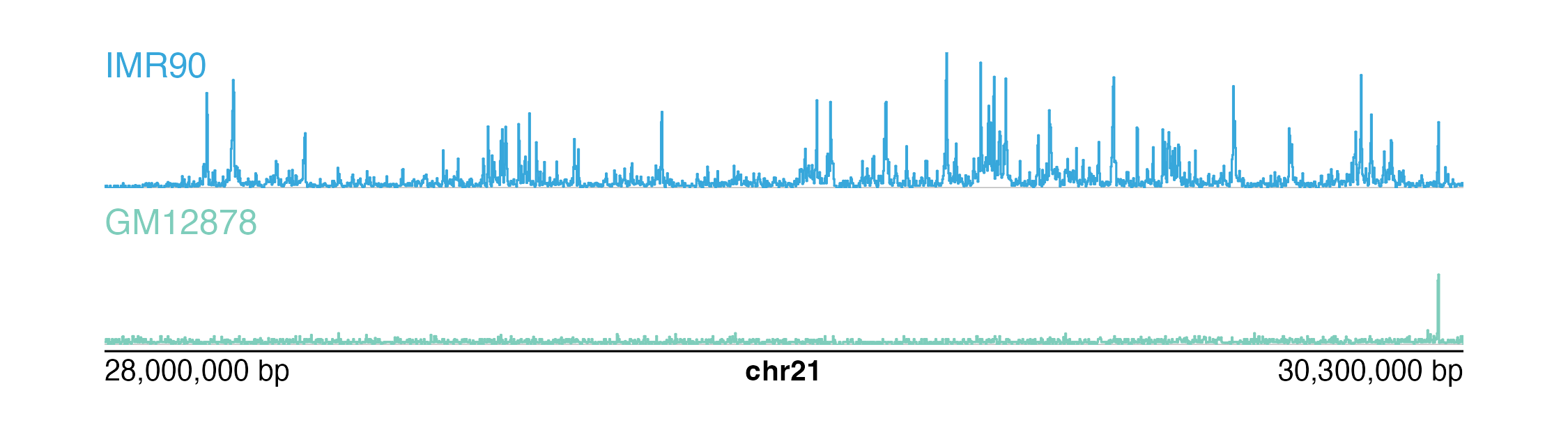plotgardener makes it easy to create reproducible, publication-quality figures from multi-omic data. Since each plot can be placed in exactly the desired location, users can stack multiple types of genomic data so that their axes and data are correctly aligned. In this section we will show some examples of plotting multi-omic data and how the pgParams object and “below” y-coordinate can facilitate this process.

In the following example, we plot the same genomic region (i.e. chr21:28000000-30300000) represented in Hi-C data, loop annotations, signal track data, GWAS data, all along a common gene track and genome label axis:

## Load example data
library(plotgardenerData)
data("IMR90_HiC_10kb")
data("IMR90_DNAloops_pairs")
data("IMR90_ChIP_H3K27ac_signal")
data("hg19_insulin_GWAS")

## Create a plotgardener page
pageCreate(
width = 3, height = 5, default.units = "inches",
showGuides = FALSE, xgrid = 0, ygrid = 0
)

## Plot Hi-C data in region
plotHicSquare(
data = IMR90_HiC_10kb,
chrom = "chr21", chromstart = 28000000, chromend = 30300000,
assembly = "hg19",
x = 0.5, y = 0.5, width = 2, height = 2,
just = c("left", "top"), default.units = "inches"
)

## Plot loop annotations
plotPairsArches(
data = IMR90_DNAloops_pairs,
chrom = "chr21", chromstart = 28000000, chromend = 30300000,
assembly = "hg19",
x = 0.5, y = 2.5, width = 2, height = 0.25,
just = c("left", "top"), default.units = "inches",
fill = "black", linecolor = "black", flip = TRUE
)

## Plot signal track data
plotSignal(
data = IMR90_ChIP_H3K27ac_signal,
chrom = "chr21", chromstart = 28000000, chromend = 30300000,
assembly = "hg19",
x = 0.5, y = 2.75, width = 2, height = 0.5,
just = c("left", "top"), default.units = "inches"
)

## Plot GWAS data
plotManhattan(
data = hg19_insulin_GWAS,
chrom = "chr21", chromstart = 28000000, chromend = 30300000,
assembly = "hg19",
ymax = 1.1, cex = 0.20,
x = 0.5, y = 3.5, width = 2, height = 0.5,
just = c("left", "top"), default.units = "inches"
)

## Plot gene track
library(TxDb.Hsapiens.UCSC.hg19.knownGene)
library(org.Hs.eg.db)
plotGenes(
chrom = "chr21", chromstart = 28000000, chromend = 30300000,
assembly = "hg19",
x = 0.5, y = 4, width = 2, height = 0.5,
just = c("left", "top"), default.units = "inches"
)

## Plot genome label
plotGenomeLabel(
chrom = "chr21", chromstart = 28000000, chromend = 30300000,
assembly = "hg19",
x = 0.5, y = 4.5, length = 2, scale = "Mb",
just = c("left", "top"), default.units = "inches"
)### Using the pgParams object

The pgParams() function creates a pgParams object that can contain any argument from plotgardener functions.

We can recreate and simplify the multi-omic plot above by saving the genomic region, left-based x-coordinate, and width into a pgParams object:

params <- pgParams(
chrom = "chr21", chromstart = 28000000, chromend = 30300000,
assembly = "hg19",
x = 0.5, just = c("left", "top"),
width = 2, length = 2, default.units = "inches"
)

Since these values are the same for each of the functions we are using to build our multi-omic figure, we can now pass the pgParams object into our functions so we don’t need to write the same parameters over and over again:

## Load example data
data("IMR90_HiC_10kb")
data("IMR90_DNAloops_pairs")
data("IMR90_ChIP_H3K27ac_signal")
data("hg19_insulin_GWAS")

## Create a plotgardener page
pageCreate(
width = 3, height = 5, default.units = "inches",
showGuides = FALSE, xgrid = 0, ygrid = 0
)

## Plot Hi-C data in region
plotHicSquare(
data = IMR90_HiC_10kb,
params = params,
y = 0.5, height = 2
)

## Plot loop annotations
plotPairsArches(
data = IMR90_DNAloops_pairs,
params = params,
y = 2.5, height = 0.25,
fill = "black", linecolor = "black", flip = TRUE
)

## Plot signal track data
plotSignal(
data = IMR90_ChIP_H3K27ac_signal,
params = params,
y = 2.75, height = 0.5
)

## Plot GWAS data
plotManhattan(
data = hg19_insulin_GWAS,
params = params,
ymax = 1.1, cex = 0.20,
y = 3.5, height = 0.5
)

## Plot gene track
library(TxDb.Hsapiens.UCSC.hg19.knownGene)
library(org.Hs.eg.db)
plotGenes(
params = params,
y = 4, height = 0.5
)

## Plot genome label
plotGenomeLabel(
params = params,
y = 4.5, scale = "Mb"
)The pgParams object also simplifies the code for making complex multi-omic figures when we want to change the genomic region of our plots. If we want to change the region for the figure above, we can simply put it into the pgParams object and re-run the code to generate the figure:

params <- pgParams(
chrom = "chr21", chromstart = 29000000, chromend = 30000000,
assembly = "hg19",
x = 0.5, just = c("left", "top"),
width = 2, length = 2, default.units = "inches"
)Alternatively, if we want to plot around a particular gene rather than a genomic region we can use pgParams() to specify gene and geneBuffer. If geneBuffer is not included, the default buffer adds (gene length) / 2 base pairs to the ends of the gene coordinates.

params <- pgParams(
gene = "LINC00113", geneBuffer = 100000, assembly = "hg19",
x = 0.5, just = c("left", "top"),
width = 2, length = 2, default.units = "inches"
)### The “below” y-coordinate

Since multi-omic plots often involve vertical stacking, the placement of multi-omic plots can be facilitated with the “below” y-coordinate. Rather than providing a numeric value or unit object to the y parameter in plotting functions, we can place a plot below the previously drawn plotgardener plot with a character value consisting of the distance below the last plot, in page units, and “b”. For example, on a page made in inches, y = "0.1b" will place a plot 0.1 inches below the last drawn plot.

We can further simplify the placement code of our multi-omic figure above by using the “below” y-coordinate to easily stack our plots:

## Load example data
data("IMR90_HiC_10kb")
data("IMR90_DNAloops_pairs")
data("IMR90_ChIP_H3K27ac_signal")
data("hg19_insulin_GWAS")

## pgParams
params <- pgParams(
chrom = "chr21", chromstart = 28000000, chromend = 30300000,
assembly = "hg19",
x = 0.5, just = c("left", "top"),
width = 2, length = 2, default.units = "inches"
)

## Create a plotgardener page
pageCreate(
width = 3, height = 5, default.units = "inches",
showGuides = FALSE, xgrid = 0, ygrid = 0
)

## Plot Hi-C data in region
plotHicSquare(
data = IMR90_HiC_10kb,
params = params,
y = 0.5, height = 2
)

## Plot loop annotations
plotPairsArches(
data = IMR90_DNAloops_pairs,
params = params,
y = "0b",
height = 0.25,
fill = "black", linecolor = "black", flip = TRUE
)

## Plot signal track data
plotSignal(
data = IMR90_ChIP_H3K27ac_signal,
params = params,
y = "0b",
height = 0.5
)

## Plot GWAS data
plotManhattan(
data = hg19_insulin_GWAS,
params = params,
ymax = 1.1, cex = 0.20,
y = "0.25b",
height = 0.5
)

## Plot gene track
library(TxDb.Hsapiens.UCSC.hg19.knownGene)
library(org.Hs.eg.db)
plotGenes(
params = params,
y = "0b",
height = 0.5
)

## Plot genome label
plotGenomeLabel(
params = params,
y = "0b",
scale = "Mb"
)### Plotting and comparing multiple signal tracks

In many multi-omic visualizations, multiple signal tracks are often aligned and stacked to compare different kinds of signal data and/or signals from different samples. plotgardener does not normalize signal data based on variables like read depth, but it is possible to scale plotgardener signal plots to the same y-axis.

To determine the appropriate y-axis range, we first must get the maximum signal score from all of our datasets to be compared:

library(plotgardenerData)
data("IMR90_ChIP_H3K27ac_signal")
data("GM12878_ChIP_H3K27ac_signal")

maxScore <- max(c(IMR90_ChIP_H3K27ac_signal$score, GM12878_ChIP_H3K27ac_signal$score))
print(maxScore)
#>  40.91454

In each of our signal plotting calls, we will then use the range parameter to set the range of both our y-axes to c(0, maxScore). Here we can do this with our pgParams object:

params <- pgParams(
chrom = "chr21",
chromstart = 28000000, chromend = 30300000,
assembly = "hg19",
range = c(0, maxScore)
)

We are now ready to plot, align, and compare our signal plots along the genomic x-axis and the score y-axis:

## Create a page
pageCreate(width = 7.5, height = 2.1, default.units = "inches",
showGuides = FALSE, xgrid = 0, ygrid = 0)

## Plot and place signal plots
signal1 <- plotSignal(
data = IMR90_ChIP_H3K27ac_signal, params = params,
x = 0.5, y = 0.25, width = 6.5, height = 0.65,
just = c("left", "top"), default.units = "inches"
)

signal2 <- plotSignal(
data = GM12878_ChIP_H3K27ac_signal, params = params,
linecolor = "#7ecdbb",
x = 0.5, y = 1, width = 6.5, height = 0.65,
just = c("left", "top"), default.units = "inches"
)

## Plot genome label
plotGenomeLabel(
chrom = "chr21",
chromstart = 28000000, chromend = 30300000,
assembly = "hg19",
x = 0.5, y = 1.68, length = 6.5,
default.units = "inches"
)

plotText(
label = "IMR90", fonsize = 10, fontcolor = "#37a7db",
x = 0.5, y = 0.25, just = c("left", "top"),
default.units = "inches"
)
plotText(
label = "GM12878", fonsize = 10, fontcolor = "#7ecdbb",
x = 0.5, y = 1, just = c("left", "top"),
default.units = "inches"
)## Session Info

sessionInfo()
#> R version 4.2.0 (2022-04-22)
#> Platform: x86_64-apple-darwin17.0 (64-bit)
#> Running under: macOS Big Sur/Monterey 10.16
#>
#> Matrix products: default
#> BLAS:   /Library/Frameworks/R.framework/Versions/4.2/Resources/lib/libRblas.0.dylib
#> LAPACK: /Library/Frameworks/R.framework/Versions/4.2/Resources/lib/libRlapack.dylib
#>
#> locale:
#>  en_US.UTF-8/en_US.UTF-8/en_US.UTF-8/C/en_US.UTF-8/en_US.UTF-8
#>
#> attached base packages:
#>  stats4    grid      stats     graphics  grDevices utils     datasets
#>  methods   base
#>
#> other attached packages:
#>   org.Hs.eg.db_3.15.0
#>   TxDb.Hsapiens.UCSC.hg19.knownGene_3.2.2
#>   GenomicFeatures_1.48.0
#>   AnnotationDbi_1.58.0
#>   Biobase_2.56.0
#>   GenomicRanges_1.48.0
#>   GenomeInfoDb_1.32.0
#>   IRanges_2.30.0
#>   S4Vectors_0.34.0
#>  BiocGenerics_0.42.0
#>  plotgardenerData_1.1.0
#>  plotgardener_1.2.0
#>
#> loaded via a namespace (and not attached):
#>   bitops_1.0-7                matrixStats_0.62.0
#>   fs_1.5.2                    bit64_4.0.5
#>   filelock_1.0.2              progress_1.2.2
#>   httr_1.4.2                  RColorBrewer_1.1-3
#>   rprojroot_2.0.3             tools_4.2.0
#>  bslib_0.3.1                 utf8_1.2.2
#>  R6_2.5.1                    DBI_1.1.2
#>  colorspace_2.0-3            prettyunits_1.1.1
#>  tidyselect_1.1.2            bit_4.0.4
#>  curl_4.3.2                  compiler_4.2.0
#>  textshaping_0.3.6           cli_3.3.0
#>  xml2_1.3.3                  desc_1.4.1
#>  DelayedArray_0.22.0         rtracklayer_1.56.0
#>  sass_0.4.1                  scales_1.2.0
#>  rappdirs_0.3.3              pkgdown_2.0.3
#>  systemfonts_1.0.4           stringr_1.4.0
#>  digest_0.6.29               Rsamtools_2.12.0
#>  yulab.utils_0.0.4           rmarkdown_2.14
#>  XVector_0.36.0              pkgconfig_2.0.3
#>  htmltools_0.5.2             MatrixGenerics_1.8.0
#>  highr_0.9                   dbplyr_2.1.1
#>  fastmap_1.1.0               rlang_1.0.2
#>  rstudioapi_0.13             RSQLite_2.2.12
#>  gridGraphics_0.5-1          jquerylib_0.1.4
#>  BiocIO_1.6.0                generics_0.1.2
#>  jsonlite_1.8.0              BiocParallel_1.30.0
#>  dplyr_1.0.9                 RCurl_1.98-1.6
#>  magrittr_2.0.3              ggplotify_0.1.0
#>  GenomeInfoDbData_1.2.8      Matrix_1.4-1
#>  Rcpp_1.0.8.3                munsell_0.5.0
#>  fansi_1.0.3                 lifecycle_1.0.1
#>  stringi_1.7.6               yaml_2.3.5
#>  SummarizedExperiment_1.26.0 zlibbioc_1.42.0
#>  BiocFileCache_2.4.0         blob_1.2.3
#>  parallel_4.2.0              crayon_1.5.1
#>  lattice_0.20-45             Biostrings_2.64.0
#>  hms_1.1.1                   KEGGREST_1.36.0
#>  knitr_1.39                  pillar_1.7.0
#>  rjson_0.2.21                biomaRt_2.52.0
#>  strawr_0.0.9                XML_3.99-0.9
#>  glue_1.6.2                  evaluate_0.15
#>  data.table_1.14.2           png_0.1-7
#>  vctrs_0.4.1                 gtable_0.3.0
#>  purrr_0.3.4                 assertthat_0.2.1
#>  cachem_1.0.6                ggplot2_3.3.5
#>  xfun_0.30                   restfulr_0.0.13
#>  ragg_1.2.2                  tibble_3.1.6
#>  GenomicAlignments_1.32.0    plyranges_1.16.0
#>  memoise_2.0.1               ellipsis_0.3.2